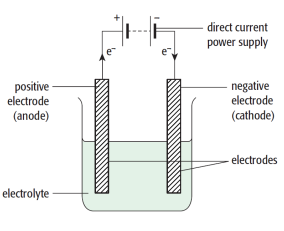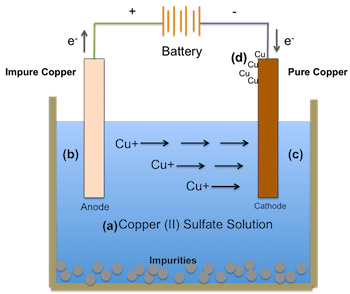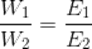Before moving into Faraday's Law of Electrolysis, let's talk about electrochemistry and some terms related to it.

Electrochemistry

The branch of chemistry that deals with the study of relationship between electrical energy (flow of electrons) and chemical reactions.Fig: Electrolytic Cell

Electrolysis ﻿﻿and Electrolytic Cell:

Electrolysis is the decomposition of a compound (electrolyte) into its constituent’s ions or radicals by an electric current and decomposition of the substance into the respective electrolytes.

The vessel or cell into which the electrolysis is carried out is called electrolytic cell. The Electrolytic cell mainly consists the following components:

1. Electrolyte : Electrolyte are those compounds which when decomposed into their respective ions or components conducts electricity in their aqueous medium. There are 2 different types of electrolyte on the basis of their ionization capability:

(a) Strong electrolytes:

These electrolytes are almost completely ionized when dissolved in a polar medium like water. In solution they are excellent conductors, e.g., HNO3

, HCl, KOH, NaOH, etc. Their degree of ionization is high and approaches unity.

(b) Weak electrolytes:

These are not completely ionized when dissolved in a polar solvent and they behave as poor conductors of electricity, e.g. CH₃COOH, H2CO3, NH4OH. Equilibrium between anions and unionized molecules is established in solution,

2. Electrodes: An electrode is an metallic conductor that carries current to an non-metallic solids,gas,liquids,vacuum .Electrodes are good conductors but they are not all metals only.

Electrode connected to positive(+) terminal of external emf or battery is called anode and electrode connected to negative(-) terminal of external emf or battery is called cathode.

It states, during electrolysis, the mass of substance (m) which is deposited at any electrode under the influence of electrical energy is proportional to the quantity of electrical charge (Q) passed through the electrolyte.

In the mathematical form, this law can be represented as follows:w α Q         w= zQ (  Where z is a proportionality constant   called Electrochemical equivalent)                                             w= zIt ( If a current of ‘I’                         Amperes is passed for a time interval of ‘t’ seconds)If we put Q = 1 coulombs in the above equation, we will get z = w which implies that electrochemical equivalent of any substance is the mass of the substance deposited on the passing of 1 coulomb through its solution.

It states that, when the same amount of electrical charge (Q) is passed through several electrolytes, the mass of the substances deposited at the respective electrodes are proportional to their respective chemical equivalent or equivalent weight.

If  W1 ,Wbe the mass of substances deposited at respective electrodes and E1 and E2 be their respective chemical equivalent or equivalent weight, then

Mathematically, Faraday’s Second law can be represented as;Sonu Kumar Patel asked almost 2 years ago

What is the difference between a simple cell and a galvanic cell?Manoj Gurung answered almost 2 years ago

Sorry for my late response. I didn't used the mattrab for a week long due to practical exam.

Simple cell is usually referred to as a cell from which chemical reaction is carried out by the help of electricity.It is also know as electrolytic cell

Where as in galvanic cell, electricity is generated out of chemical reaction(i.e. redox reaction).Galvanic cell is also know as electrochemical cell.